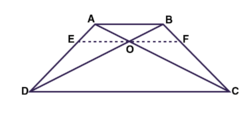# ABCD is a trapezium in which AB parallel DC and its diagonals intersect each other at point O . show that AO/BO = CO/DOGiven parameters

ABCD is a trapezium where AB || DC and diagonals AC and BD intersect at O.

To prove

$$\frac{AO}{BO} = \frac{CO}{DO}$$

Construction

Draw a line EF passing through O and also parallel to AB

Now, AB ll CD

By construction EF ll AB

∴ EF ll CD

Where EO ll AB

According to the basic proportionality theorem

$$\frac{AE}{ED} = \frac{AO}{OC}$$ ………………………………(1)

Now consider Δ ABD

where EO ll AB

According to the basic proportionality theorem

DE/EA = DO/BO…………….(ii)

From equation (i) and (ii), we get,

AO/CO = BO/DO

⇒AO/BO = CO/DO

Hence, proved.

Hence the proof.